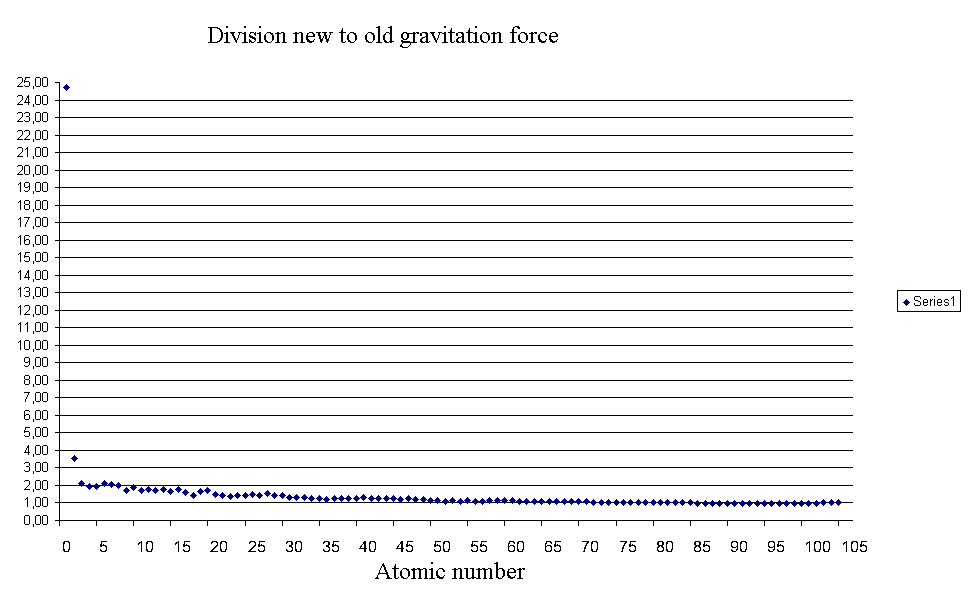HomeThe calculations were carried out with the following data here . The force was calculated with both formula for two of the same elements that a kilogram mass possess [ kg] and located at a distance of a meter [m ] of each other and the earth . Here it is unusual when compared with age- Newtonian formula that light elements attract more than the old formula states . The result of the calculation of gravitation for heavy elements is comparable to the old and new formula . The closest match of the forces I got for lead. But it is amazing deviant hydrogen, the atoms of which almost 25 times stronger dressed, but in terms of a kilogram of states than the Newtonian formula for a kilogram and a meter removal of hydrogen . The motion of cosmic objects ( in distance Millions of light years ) can only be explained when one would addiren not visible to the 4% to 5% of visible material . It is missing 95-96 % of matter that supposedly should not be visible. If the 4 % of the visible matter in the universe almost entirely of hydrogen, then you can access the " dark " entities , such as " dark matter " or " dark energy " refrain , if one were to calculate the gravitational force for my formula and not by gravitational force formula of Newton.